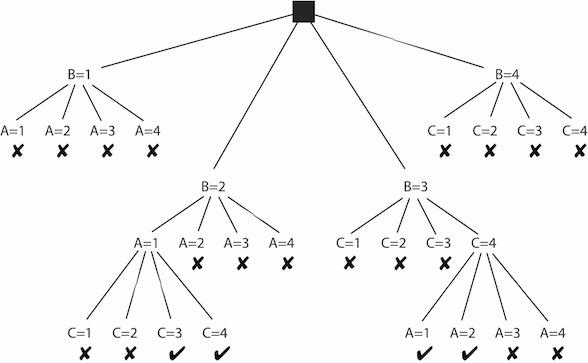## 4.4 Solving CSPs Using Search

Generate-and-test algorithms assign values to all variables before checking the constraints. Because individual constraints only involve a subset of the variables, some constraints can be tested before all of the variables have been assigned values. If a partial assignment is inconsistent with a constraint, any complete assignment that extends the partial assignment will also be inconsistent.

Example 4.12: In the delivery scheduling problem of Example 4.8, the assignments A=1 and B=1 are inconsistent with the constraint A≠B regardless of the values of the other variables. If the variables A and B are assigned values first, this inconsistency can be discovered before any values are assigned to C, D, or E, thus saving a large amount of work.

An alternative to generate-and-test algorithms is to construct a search space from which the search strategies of the previous chapter can be used. The search problem can be defined as follows:

• The nodes are assignments of values to some subset of the variables.
• The neighbors of a node N are obtained by selecting a variable V that is not assigned in node N and by having a neighbor for each assignment of a value to V that does not violate any constraint.

Suppose that node N represents the assignment X1=v1,...,Xk=vk. To find the neighbors of N, select a variable Y that is not in the set {X1,...,Xk}. For each value yi∈dom(Y), such that X1=v1,...,Xk=vk,Y=yi is consistent with the constraints, X1=v1,...,Xk=vk,Y=yi is a neighbor of N.

• The start node is the empty assignment that does not assign a value to any variables.
• A goal node is a node that assigns a value to every variable. Note that this only exists if the assignment is consistent with the constraints.

In this case, it is not the path that is of interest, but the goal nodes.

Example 4.13: Suppose you have a CSP with the variables A, B, and C, each with domain {1,2,3,4}. Suppose the constraints are A<B and B<C. A possible search tree is shown in Figure 4.1.Figure 4.1: Search tree for the CSP of Example 4.13

In this figure, a node corresponds to all of the assignments from the root to that node. The potential nodes that are pruned because they violate constraints are labeled with ✘. The leftmost ✘ corresponds to the assignment A=1, B=1. This violates the A<B constraint, and so it is pruned.

This CSP has four solutions. The leftmost one is A=1, B=2, C=3. The size of the search tree, and thus the efficiency of the algorithm, depends on which variable is selected at each time. A static ordering, such as always splitting on A then B then C, is less efficient than the dynamic ordering used here. The set of answers is the same regardless of the variable ordering.

In the preceding example, there would be 43=64 assignments tested in a generate-and-test algorithm. For the search method, there are 22 assignments generated.

Searching with a depth-first search, typically called backtracking, can be much more efficient than generate and test. Generate and test is equivalent to not checking constraints until reaching the leaves. Checking constraints higher in the tree can prune large subtrees that do not have to be searched.